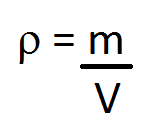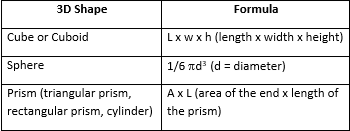# Mass and Volume: Formulas, Unit Conversion & Practice Problems

An error occurred trying to load this video.

Try refreshing the page, or contact customer support.

Coming up next: How to Find the Square Root of a Number

### You're on a roll. Keep up the good work!

Replay
Your next lesson will play in 10 seconds
• 0:00 Mass vs. Volume
• 1:12 Formulas for Mass & Volume
• 2:05 Unit Conversion
• 3:05 Practice Problems
• 5:35 Lesson Summary
Save Save

Want to watch this again later?

Timeline
Autoplay
Autoplay
Speed Speed

#### Recommended Lessons and Courses for You

Lesson Transcript
Instructor
David Wood

David has taught Honors Physics, AP Physics, IB Physics and general science courses. He has a Masters in Education, and a Bachelors in Physics.

Expert Contributor
Dawn Mills

Dawn has taught chemistry and forensic courses at the college level for 9 years. She has a PhD in Chemistry and is an author of peer reviewed publications in chemistry.

Learn about how mass and volume are connected by a special formula, how to calculate volumes of common 3D shapes, and how to convert between different units of mass and volume. Complete some practice problems and perfect your skills.

## Mass vs. Volume

Everything we've ever known or experienced is matter, and all matter has mass. The ground we walk on is matter; the air we breathe is matter; and there's even matter in space. Mass is the amount of 'stuff' inside an object; the more stuff there is, the more mass.

Matter is made up of atoms and molecules, and the more of them we have, the more the mass of an object is. We measure mass in units like kilograms, ounces, and pounds. In science, we prefer kilograms, which are the SI (Standard International) unit of mass. Your bathroom scale is calibrated to tell you your mass, but it only works properly on Earth. If you took it to the moon, the weight on the scale would change, but of course, your mass would not.

Volume is how much space something takes up. The standard unit of volume is meters cubed (or cubic meters). The classic question people ask to show the difference between mass and volume is, What has the greatest mass, a pound of feathers or a pound of nails? Well, it's a pound of each, so they both have the same mass. It's just that a pound of feathers will take up a huge amount of space; it has a greater volume.

## Formulas for Mass and Volume

Formulas are about relationships between numbers. Mass and volume relate to each other through a concept called density. Density is how tightly packed the atoms and molecules in a substance are, measured in kilograms per meter cubed. If you have a lot of mass in a small area, that's high density. If you have little mass spread over a large area, that's low density.

The formula that relates density, mass, and volume looks like this:Here, m represents the mass of an object or material, V represents the volume, and the curly p (which is the Greek letter rho) represents the density.

There are also many formulas for volume, depending on the shape of an object. The way to calculate the volume of a sphere is different than how you calculate the volume of a cube or cuboid, for example. Here is a table of some common volume formulas that you can use to solve problems:## Unit Conversion

We've already talked about the standard units of density (kilograms per meter cubed), mass (kilograms), and volume (meters cubed). But sometimes people can use non-standard or non-scientific units. For example, if someone uses a mass in grams, and a volume in centimeters cubed, then the density will come out in grams per cubic centimeter. But, sometimes you'll want to be a good scientist and use standard units instead. In those situations, it can be helpful to convert between units. You might need to multiply or divide.

Here is some information that you might need:

• There are 1000 grams in a kilogram
• There are 100 centimeters in a meter
• There are roughly 2.2 pounds in a kilogram (or 0.45 kilograms in a pound)
• There are 16 ounces in a pound
• There are 0.0823 cubic meters in a cubic foot (or 5.31 cubic feet in a cubic meter)
• There are 1000 meters in a cubic liter
• There are a million cubic centimeters in a cubic meter

## Practice Problems

Let's take a look at some examples!

#### Example 1:

A box of apples is being imported, and the density needs to be declared in kilograms per meter cubed. The apples are put on a scale, and have a mass of 15 pounds. The box is a perfect cube, and each side has a length of 0.5 meters. What is the density of the box of apples in kilograms per meter cubed?

We'll have to use the density equation, and divide mass by volume. We're told the mass is 15 pounds, but we don't know the volume. The volume of a cube is length multiplied by width multiplied by height, which is 0.5 * 0.5 * 0.5. That gives us 0.125 meters cubed.

To unlock this lesson you must be a Study.com Member.

## Materials

• Small kitchen scale
• Glass (or plastic) measuring cup
• 3 different small objects (that can fit within your glass measuring cup)
• Paper
• Pen or pencil
• Calculator

## Directions

1. Write a description of the three-dimensional objects on your sheet of paper.

2. Weigh each object on your scale and record its mass in grams.

3. Fill up the glass measuring cup to a volume that you can record and will cover your item(s) completely e.g. fill to 1/2 cup.

4. Record the initial volume of the measuring cup.

5. Place one object in the measuring cup and record the new volume. The volume can be estimated or rounded to the closest increment on the measuring cup.

6. Repeat steps 4-5 for the remaining objects.

7. Subtract the initial volume from the final volume for each item to determine their change in volume (remain in units of cups).

8. Convert the units of volume from cups to milliliters for the change in volume you calculated in step 7. The conversion factor is 1 cup = 236.59 mL.

9. Calculate the density of each item. Recall that density in the ration of the object's mass m and its volume

V.

ρ = {m}/{V}

The units should be in g/mL.

## Questions

1. Which object has the largest mass?

2. Which object has the smallest mass?

3. Which object takes up the most volume?

4. Which object takes up the least volume?

5. Which object has the largest density?

### Register to view this lesson

Are you a student or a teacher?

#### See for yourself why 30 million people use Study.com

##### Become a Study.com member and start learning now.
Back
What teachers are saying about Study.com

### Earning College Credit

Did you know… We have over 200 college courses that prepare you to earn credit by exam that is accepted by over 1,500 colleges and universities. You can test out of the first two years of college and save thousands off your degree. Anyone can earn credit-by-exam regardless of age or education level.